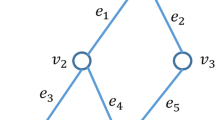# Inverse Max + Sum spanning tree problem by modifying the sum-cost vector under weighted $$l_\infty$$ Norm

## Abstract

The inverse max + sum spanning tree (IMSST) problem is studied, which is the first inverse problem on optimization problems with combined minmax–minsum objective functions. Given an edge-weighted undirected network $$G(V,E,c,w)$$, the MSST problem is to find a spanning tree $$T$$ which minimizes the combined weight $$\max _{e\in T}w(e)+\sum _{e\in T}c(e)$$, which can be solved in $$O(m\log n)$$ time, where $$m:=|E|$$ and $$n:=|V|$$. Whereas, in an IMSST problem, a spanning tree $$T_0$$ of $$G$$ is given, which is not an optimal MSST. A new sum-cost vector $$\bar{c}$$ is to be identified so that $$T_0$$ becomes an optimal MSST of the network $$G(V,E,\bar{c},w)$$, where $$0\le c-l\le \bar{c} \le c+u$$ and $$l,u\ge 0$$. The objective is to minimize the cost $$\max _{e\in E}q(e)|\bar{c}(e)-c(e)|$$ incurred by modifying the sum-cost vector $$c$$ under weighted $$l_\infty$$ norm, where $$q(e)\ge 1$$. We show that the unbounded IMSST problem is a linear fractional combinatorial optimization (LFCO) problem and develop a discrete type Newton method to solve it. Furthermore, we prove an $$O(m)$$ bound on the number of iterations, although most LFCO problems can be solved in $$O(m^2 \log m)$$ iterations. Therefore, both the unbounded and bounded IMSST problems can be solved by solving $$O(m)$$ MSST problems. Computational results show that the algorithms can efficiently solve the problems.

This is a preview of subscription content, log in via an institution to check access.

Price excludes VAT (USA)
Tax calculation will be finalised during checkout.

### Similar content being viewed by others### Inverse max+sum spanning tree problem under weighted $$l_{\infty }$$ norm by modifying max-weight vector

Junhua Jia, Xiucui Guan, … Panos M. Pardalos### Inverse max+sum spanning tree problem under weighted $$l_1$$ norm by modifying the sum-cost vector

Xiucui Guan, Panos M. Pardalos & Binwu Zhang

### Inverse max  $$+$$  sum spanning tree problem under Hamming distance by modifying the sum-cost vector

Xiucui Guan, Xinyan He, … Binwu Zhang

## References

1. Ahuja, R.K., Orlin, J.B.: A faster algorithm for the inverse spanning tree problem. J. Algorithms 34, 177–193 (2000)

2. Duin, C.W., Volgenant, A.: Minimum deviation and balanced optimization: a unified approach. Oper. Res. Lett. 10, 43–48 (1991)

3. Duin, C.W., Volgenant, A.: Some inverse optimization problems under the Hamming distance. Eur. J. Oper. Res. 170, 887–899 (2006)

4. Guan, X.C., Zhang, J.Z.: Inverse constrained bottleneck problems under weighted $$l_\infty$$ norm. Comput. Oper. Res. 34, 3243–3254 (2007)

5. He, Y., Zhang, B., Yao, E.: Weighted inverse minimum spanning tree problems under Hamming distance. J. Comb. Optim. 9, 91–100 (2005)

6. Heuburger, C.: Inverse optimization: a survey on problems, methods, and results. J. Comb. Optim. 8(3), 329–361 (2004)

7. Hochbaum, D.S.: Efficient algorithms for the inverse spanning tree problem. Oper. Res. 51(5), 785–797 (2003)

8. Liu, L.C., Wang, Q.: Constrained inverse min–max spanning tree problems under the weighted Hamming distance. J. Glob. Optim. 43, 83–95 (2009)

9. Minoux, M.: Solving combinatorial problems with combined minmax–minsum objective and applications. Math. Program. (Ser. B) 45, 361–371 (1989)

10. Punnen, A.P.: On combined minmax–minsum optimization. Comput. Oper. Res. 21(6), 707–716 (1994)

11. Punnen, A.P., Nair, K.P.K.: An $$O(m \log n)$$ algorithm for the max + sum spanning tree problem. Eur. J. Oper. Res. 89, 423–426 (1996)

12. Radzik, T.: Parametric flows, weighted means of cuts, and fractional combinatorial optimization. In: Pardalos, P.M. (ed.) Complexity in Numerical Optimization, pp. 351–386. World Scientific, Singapore (1993)

13. Roland, J., Figueira, J.R., Smet, Y.D.: The inverse $$\{0,1\}$$-knapsack problem: theory, algorithms and computational experiments. Discret. Optim. 10, 181–192 (2013)

14. Scheinerman, E.R.: Matgraph is a Matlab Toolbox for Simple Graphs. http://www.ams.jhu.edu/~ers/matgraph/

15. Sokkalingam, P.T., Ahuja, R.K., Orlin, J.B.: Solving inverse spanning tree problems through network flow techniques. Oper. Res. 47(2), 291–298 (1999)

16. Wang, Q., Yang, X.G., Zhang, J.Z.: A class of inverse dominant problems under weighted $$l_\infty$$ norm and an improved complexity bound for Radziks algorithm. J. Glob. Optim. 34, 551–567 (2006)

17. Yamada, T., Takahashi, H., Kataoka, S.: A heuristic algorithm for the mini–max spanning forest problem. Eur. J. Oper. Res. 91, 565–572 (1996)

18. Yang, C., Zhang, J.: Two general methods for inverse optimization problems. Appl. Math. Lett. 12, 69–72 (1999)

19. Yang, X.G., Zhang, J.Z.: Some inverse min–max network problems under weighted $$l_1$$ and $$l_\infty$$ norms with bound constraints on changes. J. Comb. Optim. 13, 123–135 (2007)

20. Zhang, B., Zhang, J., He, Y.: Constrained inverse minimum spanning tree problems under the bottleneck-type Hamming distance. J. Glob. Optim. 34(3), 467–474 (2006)

21. Zhang, J.Z., Liu, Z.: A general model of some inverse combinatorial optimization problems and its solution method under $$l_{\infty }$$ norm. J. Comb. Optim. 6, 207–227 (2002)

## Acknowledgments

Research is supported by National Natural Science Foundation of China (10801031) and the State Scholarship Fund of China (201208320355).

## Author information

Authors

### Corresponding author

Correspondence to Xiucui Guan.

## Rights and permissions

Reprints and Permissions

Guan, X., Pardalos, P.M. & Zuo, X. Inverse Max + Sum spanning tree problem by modifying the sum-cost vector under weighted $$l_\infty$$ Norm. J Glob Optim 61, 165–182 (2015). https://doi.org/10.1007/s10898-014-0140-z